# Thirteen ways of looking at a turtle

This post is part of the F# Advent Calendar in English 2015 project. Check out all the other great posts there! And special thanks to Sergey Tihon for organizing this.

I was discussing how to implement a simple turtle graphics system some time ago, and it struck me that, because the turtle requirements are so simple and so well known, it would make a great basis for demonstrating a range of different techniques.

So, in this two part mega-post, I'll stretch the turtle model to the limit while demonstrating things like: partial application, validation with Success/Failure results, the concept of "lifting", agents with message queues, dependency injection, the State monad, event sourcing, stream processing, and finally a custom interpreter!

Without further ado then, I hereby present thirteen different ways of implementing a turtle:

and 2 bonus ways for the extended edition:

All source code for this post is available on github.

## The requirements for a Turtle

A turtle supports four instructions:

• Move some distance in the current direction.
• Turn a certain number of degrees clockwise or anticlockwise.
• Put the pen down or up. When the pen is down, moving the turtle draws a line.
• Set the pen color (one of black, blue or red).

These requirements lead naturally to some kind of "turtle interface" like this:

• `Move aDistance`
• `Turn anAngle`
• `PenUp`
• `PenDown`
• `SetColor aColor`

All of the following implementations will be based on this interface or some variant of it.

Note that the turtle must convert these instructions to drawing lines on a canvas or other graphics context. So the implementation will probably need to keep track of the turtle position and current state somehow.

## Common code

Before we start implementing, let's get some common code out of the way.

First, we'll need some types to represent distances, angles, the pen state, and the pen colors.

``````/// An alias for a float
type Distance = float

/// Use a unit of measure to make it clear that the angle is in degrees, not radians
type [<Measure>] Degrees

/// An alias for a float of Degrees
type Angle  = float<Degrees>

/// Enumeration of available pen states
type PenState = Up | Down

/// Enumeration of available pen colors
type PenColor = Black | Red | Blue
``````

and we'll also need a type to represent the position of the turtle:

``````/// A structure to store the (x,y) coordinates
type Position = {x:float; y:float}
``````

We'll also need a helper function to calculate a new position based on moving a certain distance at a certain angle:

``````// round a float to two places to make it easier to read
let round2 (flt:float) = Math.Round(flt,2)

/// calculate a new position from the current position given an angle and a distance
let calcNewPosition (distance:Distance) (angle:Angle) currentPos =
// Convert degrees to radians with 180.0 degrees = 1 pi radian
let angleInRads = angle * (Math.PI/180.0) * 1.0<1/Degrees>
// current pos
let x0 = currentPos.x
let y0 = currentPos.y
// new pos
let x1 = x0 + (distance * cos angleInRads)
let y1 = y0 + (distance * sin angleInRads)
// return a new Position
{x=round2 x1; y=round2 y1}
``````

Let's also define the initial state of a turtle:

``````/// Default initial state
let initialPosition,initialColor,initialPenState =
{x=0.0; y=0.0}, Black, Down
``````

And a helper that pretends to draw a line on a canvas:

``````let dummyDrawLine log oldPos newPos color =
// for now just log it
log (sprintf "...Draw line from (%0.1f,%0.1f) to (%0.1f,%0.1f) using %A" oldPos.x oldPos.y newPos.x newPos.y color)
``````

Now we're ready for the first implementation!

## 1. Basic OO -- A class with mutable state

In this first design, we will use an object-oriented approach and represent the turtle with a simple class.

• The state will be stored in local fields (`currentPosition`, `currentAngle`, etc) that are mutable.
• We will inject a logging function `log` so that we can monitor what happens.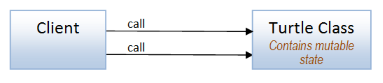And here's the complete code, which should be self-explanatory:

``````type Turtle(log) =

let mutable currentPosition = initialPosition
let mutable currentAngle = 0.0<Degrees>
let mutable currentColor = initialColor
let mutable currentPenState = initialPenState

member this.Move(distance) =
log (sprintf "Move %0.1f" distance)
// calculate new position
let newPosition = calcNewPosition distance currentAngle currentPosition
// draw line if needed
if currentPenState = Down then
dummyDrawLine log currentPosition newPosition currentColor
// update the state
currentPosition <- newPosition

member this.Turn(angle) =
log (sprintf "Turn %0.1f" angle)
// calculate new angle
let newAngle = (currentAngle + angle) % 360.0<Degrees>
// update the state
currentAngle <- newAngle

member this.PenUp() =
log "Pen up"
currentPenState <- Up

member this.PenDown() =
log "Pen down"
currentPenState <- Down

member this.SetColor(color) =
log (sprintf "SetColor %A" color)
currentColor <- color
``````

### Calling the turtle object

The client code instantiates the turtle and talks to it directly:

``````/// Function to log a message
let log message =
printfn "%s" message

let drawTriangle() =
let turtle = Turtle(log)
turtle.Move 100.0
turtle.Turn 120.0<Degrees>
turtle.Move 100.0
turtle.Turn 120.0<Degrees>
turtle.Move 100.0
turtle.Turn 120.0<Degrees>
// back home at (0,0) with angle 0
``````

The logged output of `drawTriangle()` is:

``````Move 100.0
...Draw line from (0.0,0.0) to (100.0,0.0) using Black
Turn 120.0
Move 100.0
...Draw line from (100.0,0.0) to (50.0,86.6) using Black
Turn 120.0
Move 100.0
...Draw line from (50.0,86.6) to (0.0,0.0) using Black
Turn 120.0
``````

Similarly, here's the code to draw a polygon:

``````let drawPolygon n =
let angle = 180.0 - (360.0/float n)
let angleDegrees = angle * 1.0<Degrees>
let turtle = Turtle(log)

// define a function that draws one side
let drawOneSide() =
turtle.Move 100.0
turtle.Turn angleDegrees

// repeat for all sides
for i in [1..n] do
drawOneSide()
``````

Note that `drawOneSide()` does not return anything -- all the code is imperative and stateful. Compare this to the code in the next example, which takes a pure functional approach.

• It's very easy to implement and understand.

• The stateful code is harder to test. We have to put an object into a known state state before testing, which is simple in this case, but can be long-winded and error-prone for more complex objects.
• The client is coupled to a particular implementation. No interfaces here! We'll look at using interfaces shortly.

The source code for this version is available here (turtle class) and here (client).

## 2: Basic FP - A module of functions with immutable state

The next design will use a pure, functional approach. An immutable `TurtleState` is defined, and then the various turtle functions accept a state as input and return a new state as output.

In this approach then, the client is responsible for keeping track of the current state and passing it into the next function call.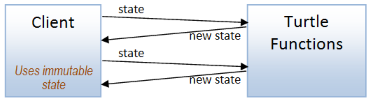Here's the definition of `TurtleState` and the values for the initial state:

``````module Turtle =

type TurtleState = {
position : Position
angle : float<Degrees>
color : PenColor
penState : PenState
}

let initialTurtleState = {
position = initialPosition
angle = 0.0<Degrees>
color = initialColor
penState = initialPenState
}
``````

And here are the "api" functions, all of which take a state parameter and return a new state:

``````module Turtle =

// [state type snipped]

let move log distance state =
log (sprintf "Move %0.1f" distance)
// calculate new position
let newPosition = calcNewPosition distance state.angle state.position
// draw line if needed
if state.penState = Down then
dummyDrawLine log state.position newPosition state.color
// update the state
{state with position = newPosition}

let turn log angle state =
log (sprintf "Turn %0.1f" angle)
// calculate new angle
let newAngle = (state.angle + angle) % 360.0<Degrees>
// update the state
{state with angle = newAngle}

let penUp log state =
log "Pen up"
{state with penState = Up}

let penDown log state =
log "Pen down"
{state with penState = Down}

let setColor log color state =
log (sprintf "SetColor %A" color)
{state with color = color}
``````

Note that the `state` is always the last parameter -- this makes it easier to use the "piping" idiom.

### Using the turtle functions

The client now has to pass in both the `log` function and the `state` to every function, every time!

We can eliminate the need to pass in the log function by using partial application to create new versions of the functions with the logger baked in:

``````/// Function to log a message
let log message =
printfn "%s" message

// versions with log baked in (via partial application)
let move = Turtle.move log
let turn = Turtle.turn log
let penDown = Turtle.penDown log
let penUp = Turtle.penUp log
let setColor = Turtle.setColor log
``````

With these simpler versions, the client can just pipe the state through in a natural way:

``````let drawTriangle() =
Turtle.initialTurtleState
|> move 100.0
|> turn 120.0<Degrees>
|> move 100.0
|> turn 120.0<Degrees>
|> move 100.0
|> turn 120.0<Degrees>
// back home at (0,0) with angle 0
``````

When it comes to drawing a polygon, it's a little more complicated, as we have to "fold" the state through the repetitions for each side:

``````let drawPolygon n =
let angle = 180.0 - (360.0/float n)
let angleDegrees = angle * 1.0<Degrees>

// define a function that draws one side
let oneSide state sideNumber =
state
|> move 100.0
|> turn angleDegrees

// repeat for all sides
[1..n]
|> List.fold oneSide Turtle.initialTurtleState
``````

• Again, it's very easy to implement and understand.
• The stateless functions are easier to test. We always provide the current state as input, so there is no setup needed to get an object into a known state.
• Because there is no global state, the functions are modular and can be reused in other contexts (as we'll see later in this post).

• As before, the client is coupled to a particular implementation.
• The client has to keep track of the state (but some solutions to make this easier are shown later in this post).

The source code for this version is available here (turtle functions) and here (client).

## 3: An API with a object-oriented core

Let's hide the client from the implementation using an API!

In this case, the API will be string based, with text commands such as `"move 100"` or `"turn 90"`. The API must validate these commands and turn them into method calls on the turtle (we'll use the OO approach of a stateful `Turtle` class again).If the command is not valid, the API must indicate that to the client. Since we are using an OO approach, we'll do this by throwing a `TurtleApiException` containing a string, like this.

``````exception TurtleApiException of string
``````

Next we need some functions that validate the command text:

``````// convert the distance parameter to a float, or throw an exception
let validateDistance distanceStr =
try
float distanceStr
with
| ex ->
let msg = sprintf "Invalid distance '%s' [%s]" distanceStr  ex.Message
raise (TurtleApiException msg)

// convert the angle parameter to a float<Degrees>, or throw an exception
let validateAngle angleStr =
try
(float angleStr) * 1.0<Degrees>
with
| ex ->
let msg = sprintf "Invalid angle '%s' [%s]" angleStr ex.Message
raise (TurtleApiException msg)

// convert the color parameter to a PenColor, or throw an exception
let validateColor colorStr =
match colorStr with
| "Black" -> Black
| "Blue" -> Blue
| "Red" -> Red
| _ ->
let msg = sprintf "Color '%s' is not recognized" colorStr
raise (TurtleApiException msg)
``````

With these in place, we can create the API.

The logic for parsing the command text is to split the command text into tokens and then match the first token to `"move"`, `"turn"`, etc.

Here's the code:

``````type TurtleApi() =

let turtle = Turtle(log)

member this.Exec (commandStr:string) =
let tokens = commandStr.Split(' ') |> List.ofArray |> List.map trimString
match tokens with
| [ "Move"; distanceStr ] ->
let distance = validateDistance distanceStr
turtle.Move distance
| [ "Turn"; angleStr ] ->
let angle = validateAngle angleStr
turtle.Turn angle
| [ "Pen"; "Up" ] ->
turtle.PenUp()
| [ "Pen"; "Down" ] ->
turtle.PenDown()
| [ "SetColor"; colorStr ] ->
let color = validateColor colorStr
turtle.SetColor color
| _ ->
let msg = sprintf "Instruction '%s' is not recognized" commandStr
raise (TurtleApiException msg)
``````

### Using the API

Here's how `drawPolygon` is implemented using the `TurtleApi` class:

``````let drawPolygon n =
let angle = 180.0 - (360.0/float n)
let api = TurtleApi()

// define a function that draws one side
let drawOneSide() =
api.Exec "Move 100.0"
api.Exec (sprintf "Turn %f" angle)

// repeat for all sides
for i in [1..n] do
drawOneSide()
``````

You can see that the code is quite similar to the earlier OO version, with the direct call `turtle.Move 100.0` being replaced with the indirect API call `api.Exec "Move 100.0"`.

Now if we trigger an error with a bad command such as `api.Exec "Move bad"`, like this:

``````let triggerError() =
let api = TurtleApi()
``````

then the expected exception is thrown:

``````Exception of type 'TurtleApiException' was thrown.
``````

What are the advantages and disadvantages of an API layer like this?

• The turtle implementation is now hidden from the client.
• An API at a service boundary supports validation and can be extended to support monitoring, internal routing, load balancing, etc.

• The API is coupled to a particular implementation, even though the client isn't.
• The system is very stateful. Even though the client does not know about the implementation behind the API, the client is still indirectly coupled to the inner core via shared state which in turn can make testing harder.

The source code for this version is available here.

## 4: An API with a functional core

An alternative approach for this scenario is to use a hybrid design, where the core of the application consists of pure functions, while the boundaries are imperative and stateful.

This approach has been named "Functional Core/Imperative Shell" by Gary Bernhardt.

Applied to our API example, the API layer uses only pure turtle functions, but the API layer manages the state (rather than the client) by storing a mutable turtle state.

Also, to be more functional, the API will not throw exceptions if the command text is not valid, but instead will return a `Result` value with `Success` and `Failure` cases, where the `Failure` case is used for any errors. (See my talk on the functional approach to error handling for a more in depth discussion of this technique).Let's start by implementing the API class. This time it contains a `mutable` turtle state:

``````type TurtleApi() =

let mutable state = initialTurtleState

/// Update the mutable state value
state <- newState
``````

The validation functions no longer throw an exception, but return `Success` or `Failure`:

``````let validateDistance distanceStr =
try
Success (float distanceStr)
with
| ex ->
Failure (InvalidDistance distanceStr)
``````

The error cases are documented in their own type:

``````type ErrorMessage =
| InvalidDistance of string
| InvalidAngle of string
| InvalidColor of string
| InvalidCommand of string
``````

Now because the validation functions now return a `Result<Distance>` rather than a "raw" distance, the `move` function needs to be lifted to the world of `Results`, as does the current state.

There are three functions that we will use when working with `Result`s: `returnR`, `mapR` and `lift2R`.

• `returnR` transforms a "normal" value into a value in the world of Results: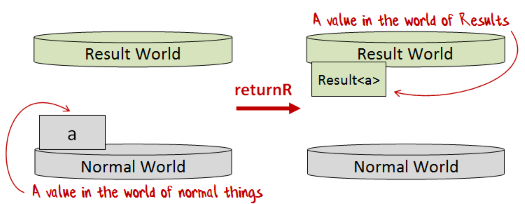• `mapR` transforms a "normal" one-parameter function into a one-parameter function in the world of Results: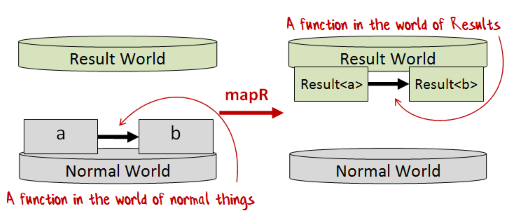• `lift2R` transforms a "normal" two-parameter function into a two-parameter function in the world of Results: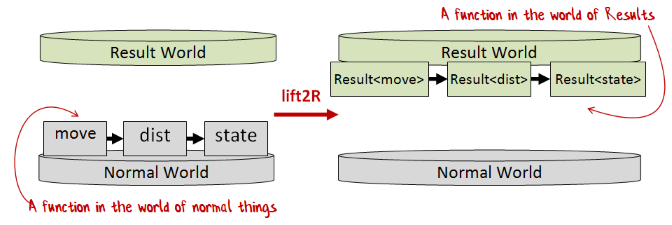As an example, with these helper functions, we can turn the normal `move` function into a function in the world of Results:

• The distance parameter is already in `Result` world
• The state parameter is lifted into `Result` world using `returnR`
• The `move` function is lifted into `Result` world using `lift2R`
``````// lift current state to Result
let stateR = returnR state

// get the distance as a Result
let distanceR = validateDistance distanceStr

// call "move" lifted to the world of Results
lift2R move distanceR stateR
``````

(For more details on lifting functions to `Result` world, see the post on "lifting" in general )

Here's the complete code for `Exec`:

``````/// Execute the command string, and return a Result
/// Exec : commandStr:string -> Result<unit,ErrorMessage>
member this.Exec (commandStr:string) =
let tokens = commandStr.Split(' ') |> List.ofArray |> List.map trimString

// lift current state to Result
let stateR = returnR state

// calculate the new state
let newStateR =
match tokens with
| [ "Move"; distanceStr ] ->
// get the distance as a Result
let distanceR = validateDistance distanceStr

// call "move" lifted to the world of Results
lift2R move distanceR stateR

| [ "Turn"; angleStr ] ->
let angleR = validateAngle angleStr
lift2R turn angleR stateR

| [ "Pen"; "Up" ] ->
returnR (penUp state)

| [ "Pen"; "Down" ] ->
returnR (penDown state)

| [ "SetColor"; colorStr ] ->
let colorR = validateColor colorStr
lift2R setColor colorR stateR

| _ ->
Failure (InvalidCommand commandStr)

// Lift `updateState` into the world of Results and
// call it with the new state.

// Return the final result (output of updateState)
``````

### Using the API

The API returns a `Result`, so the client can no longer call each function in sequence, as we need to handle any errors coming from a call and abandon the rest of the steps.

To make our lives easier, we'll use a `result` computation expression (or workflow) to chain the calls and preserve the imperative "feel" of the OO version.

``````let drawTriangle() =
let api = TurtleApi()
result {
do! api.Exec "Move 100"
do! api.Exec "Turn 120"
do! api.Exec "Move 100"
do! api.Exec "Turn 120"
do! api.Exec "Move 100"
do! api.Exec "Turn 120"
}
``````

The source code for the `result` computation expression is available here.

Similarly, for the `drawPolygon` code, we can create a helper to draw one side and then call it `n` times inside a `result` expression.

``````let drawPolygon n =
let angle = 180.0 - (360.0/float n)
let api = TurtleApi()

// define a function that draws one side
let drawOneSide() = result {
do! api.Exec "Move 100.0"
do! api.Exec (sprintf "Turn %f" angle)
}

// repeat for all sides
result {
for i in [1..n] do
do! drawOneSide()
}
``````

The code looks imperative, but is actually purely functional, as the returned `Result` values are being handled transparently by the `result` workflow.

• The same as for the OO version of an API -- the turtle implementation is hidden from the client, validation can be done, etc.
• The only stateful part of the system is at the boundary. The core is stateless which makes testing easier.

• The API is still coupled to a particular implementation.

The source code for this version is available here (api helper functions) and here (API and client).

## 5: An API in front of an agent

In this design, an API layer communicates with a `TurtleAgent` via a message queue and the client talks to the API layer as before.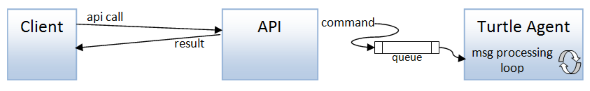There are no mutables in the API (or anywhere). The `TurtleAgent` manages state by storing the current state as a parameter in the recursive message processing loop.

Now because the `TurtleAgent` has a typed message queue, where all messages are the same type, we must combine all possible commands into a single discriminated union type (`TurtleCommand`).

``````type TurtleCommand =
| Move of Distance
| Turn of Angle
| PenUp
| PenDown
| SetColor of PenColor
``````

The agent implementation is similar to the previous ones, but rather than exposing the turtle functions directly, we now do pattern matching on the incoming command to decide which function to call:

``````type TurtleAgent() =

/// Function to log a message
let log message =
printfn "%s" message

// logged versions
let move = Turtle.move log
let turn = Turtle.turn log
let penDown = Turtle.penDown log
let penUp = Turtle.penUp log
let setColor = Turtle.setColor log

let mailboxProc = MailboxProcessor.Start(fun inbox ->
let rec loop turtleState = async {
// read a command message from teh queue
// create a new state from handling the message
let newState =
match command with
| Move distance ->
move distance turtleState
| Turn angle ->
turn angle turtleState
| PenUp ->
penUp turtleState
| PenDown ->
penDown turtleState
| SetColor color ->
setColor color turtleState
return! loop newState
}
loop Turtle.initialTurtleState )

// expose the queue externally
member this.Post(command) =
mailboxProc.Post command
``````

### Sending a command to the Agent

The API calls the agent by constructing a `TurtleCommand` and posting it to the agent's queue.

This time, rather than using the previous approach of "lifting" the `move` command:

``````let stateR = returnR state
let distanceR = validateDistance distanceStr
lift2R move distanceR stateR
``````

we'll use the `result` computation expression instead, so the code above would have looked like this:

``````result {
let! distance = validateDistance distanceStr
move distance state
}
``````

In the agent implementation, we are not calling a `move` command, but instead creating the `Move` case of the `Command` type, so the code looks like:

``````result {
let! distance = validateDistance distanceStr
let command = Move distance
turtleAgent.Post command
}
``````

Here's the complete code:

``````member this.Exec (commandStr:string) =
let tokens = commandStr.Split(' ') |> List.ofArray |> List.map trimString

// calculate the new state
let result =
match tokens with
| [ "Move"; distanceStr ] -> result {
let! distance = validateDistance distanceStr
let command = Move distance
turtleAgent.Post command
}

| [ "Turn"; angleStr ] -> result {
let! angle = validateAngle angleStr
let command = Turn angle
turtleAgent.Post command
}

| [ "Pen"; "Up" ] -> result {
let command = PenUp
turtleAgent.Post command
}

| [ "Pen"; "Down" ] -> result {
let command = PenDown
turtleAgent.Post command
}

| [ "SetColor"; colorStr ] -> result {
let! color = validateColor colorStr
let command = SetColor color
turtleAgent.Post command
}

| _ ->
Failure (InvalidCommand commandStr)

// return any errors
result
``````

• A great way to protect mutable state without using locks.
• The API is decoupled from a particular implementation via the message queue. The `TurtleCommand` acts as a sort of protocol that decouples the two ends of the queue.
• The turtle agent is naturally asynchronous.
• Agents can easily be scaled horizontally.

• Agents are stateful and have the same problem as stateful objects:
• Testing is harder.
• It is all too easy to create a web of complex dependencies between actors.
• A robust implementation for agents can get quite complex, as you may need support for supervisors, heartbeats, back pressure, etc.

The source code for this version is available here .

## 6: Dependency injection using interfaces

All the implementations so far have been tied to a specific implementation of the turtle functions, with the exception of the Agent version, where the API communicated indirectly via a queue.

So let's now look at some ways of decoupling the API from the implementation.

### Designing an interface, object-oriented style

We'll start with the classic OO way of decoupling implementations: using interfaces.

Applying that approach to the turtle domain, we can see that our API layer will need to communicate with a `ITurtle` interface rather than a specific turtle implementation. The client injects the turtle implementation later, via the API's constructor.

Here's the interface definition:

``````type ITurtle =
abstract Move : Distance -> unit
abstract Turn : Angle -> unit
abstract PenUp : unit -> unit
abstract PenDown : unit -> unit
abstract SetColor : PenColor -> unit
``````

Note that there are a lot of `unit`s in these functions. A `unit` in a function signature implies side effects, and indeed the `TurtleState` is not used anywhere, as this is a OO-based approach where the mutable state is encapsulated in the object.

Next, we need to change the API layer to use the interface by injecting it in the constructor for `TurtleApi`. Other than that, the rest of the API code is unchanged, as shown by the snippet below:

``````type TurtleApi(turtle: ITurtle) =

// other code

member this.Exec (commandStr:string) =
let tokens = commandStr.Split(' ') |> List.ofArray |> List.map trimString
match tokens with
| [ "Move"; distanceStr ] ->
let distance = validateDistance distanceStr
turtle.Move distance
| [ "Turn"; angleStr ] ->
let angle = validateAngle angleStr
turtle.Turn angle
// etc
``````

### Creating some implementations of an OO interface

Now let's create and test some implementations.

The first implementation will be called `normalSize` and will be the original one. The second will be called `halfSize` and will reduce all the distances by half.

For `normalSize` we could go back and retrofit the orginal `Turtle` class to support the `ITurtle` interface. But I hate having to change working code! Instead, we can create a "proxy" wrapper around the orginal `Turtle` class, where the proxy implements the new interface.

In some languages, creating proxy wrappers can be long-winded, but in F# you can use object expressions to implement an interface quickly:

``````let normalSize() =
let log = printfn "%s"
let turtle = Turtle(log)

// return an interface wrapped around the Turtle
{new ITurtle with
member this.Move dist = turtle.Move dist
member this.Turn angle = turtle.Turn angle
member this.PenUp() = turtle.PenUp()
member this.PenDown() = turtle.PenDown()
member this.SetColor color = turtle.SetColor color
}
``````

And to create the `halfSize` version, we do the same thing, but intercept the calls to `Move` and halve the distance parameter:

``````let halfSize() =
let normalSize = normalSize()

// return a decorated interface
{new ITurtle with
member this.Move dist = normalSize.Move (dist/2.0)   // halved!!
member this.Turn angle = normalSize.Turn angle
member this.PenUp() = normalSize.PenUp()
member this.PenDown() = normalSize.PenDown()
member this.SetColor color = normalSize.SetColor color
}
``````

This is actually the "decorator" pattern at work: we're wrapping `normalSize` in a proxy with an identical interface, then changing the behavior for some of the methods, while passing others though untouched.

### Injecting dependencies, OO style

Now let's look at the client code that injects the dependencies into the API.

First, some code to draw a triangle, where a `TurtleApi` is passed in:

``````let drawTriangle(api:TurtleApi) =
api.Exec "Move 100"
api.Exec "Turn 120"
api.Exec "Move 100"
api.Exec "Turn 120"
api.Exec "Move 100"
api.Exec "Turn 120"
``````

And now let's try drawing the triangle by instantiating the API object with the normal interface:

``````let iTurtle = normalSize()   // an ITurtle type
let api = TurtleApi(iTurtle)
drawTriangle(api)
``````

Obviously, in a real system, the dependency injection would occur away from the call site, using an IoC container or similar.

If we run it, the output of `drawTriangle` is just as before:

``````Move 100.0
...Draw line from (0.0,0.0) to (100.0,0.0) using Black
Turn 120.0
Move 100.0
...Draw line from (100.0,0.0) to (50.0,86.6) using Black
Turn 120.0
Move 100.0
...Draw line from (50.0,86.6) to (0.0,0.0) using Black
Turn 120.0
``````

And now with the half-size interface..

``````let iTurtle = halfSize()
let api = TurtleApi(iTurtle)
drawTriangle(api)
``````

...the output is, as we hoped, half the size!

``````Move 50.0
...Draw line from (0.0,0.0) to (50.0,0.0) using Black
Turn 120.0
Move 50.0
...Draw line from (50.0,0.0) to (25.0,43.3) using Black
Turn 120.0
Move 50.0
...Draw line from (25.0,43.3) to (0.0,0.0) using Black
Turn 120.0
``````

### Designing an interface, functional style

In a pure FP world, OO-style interfaces do not exist. However, you can emulate them by using a record containing functions, with one function for each method in the interface.

So let's create a alternative version of dependency injection, where this time the API layer will use a record of functions rather than an interface.

A record of functions is a normal record, but the types of the fields are function types. Here's the definition we'll use:

``````type TurtleFunctions = {
move : Distance -> TurtleState -> TurtleState
turn : Angle -> TurtleState -> TurtleState
penUp : TurtleState -> TurtleState
penDown : TurtleState -> TurtleState
setColor : PenColor -> TurtleState -> TurtleState
}
``````

Note that there are no `unit`s in these function signatures, unlike the OO version. Instead, the `TurtleState` is explicitly passed in and returned.

Also note that there is no logging either. The logging method will be baked in to the functions when the record is created.

The `TurtleApi` constructor now takes a `TurtleFunctions` record rather than an `ITurtle`, but as these functions are pure, the API needs to manage the state again with a `mutable` field.

``````type TurtleApi(turtleFunctions: TurtleFunctions) =

let mutable state = initialTurtleState
``````

The implementation of the main `Exec` method is very similar to what we have seen before, with these differences:

• The function is fetched from the record (e.g. `turtleFunctions.move`).
• All the activity takes place in a `result` computation expression so that the result of the validations can be used.

Here's the code:

``````member this.Exec (commandStr:string) =
let tokens = commandStr.Split(' ') |> List.ofArray |> List.map trimString

// return Success of unit, or Failure
match tokens with
| [ "Move"; distanceStr ] -> result {
let! distance = validateDistance distanceStr
let newState = turtleFunctions.move distance state
}
| [ "Turn"; angleStr ] -> result {
let! angle = validateAngle angleStr
let newState = turtleFunctions.turn angle state
}
// etc
``````

### Creating some implementations of a "record of functions"

Noe let's create some implementations.

Again, we'll have a `normalSize` implementation and a `halfSize` implementation.

For `normalSize` we just need to use the functions from the original `Turtle` module, with the logging baked in using partial application:

``````let normalSize() =
let log = printfn "%s"
// return a record of functions
{
move = Turtle.move log
turn = Turtle.turn log
penUp = Turtle.penUp log
penDown = Turtle.penDown log
setColor = Turtle.setColor log
}
``````

And to create the `halfSize` version, we clone the record, and change just the `move` function:

``````let halfSize() =
let normalSize = normalSize()
// return a reduced turtle
{ normalSize with
move = fun dist -> normalSize.move (dist/2.0)
}
``````

What's nice about cloning records rather than proxying interfaces is that we don't have to reimplement every function in the record, just the ones we care about.

### Injecting dependencies again

The client code that injects the dependencies into the API is implemented just as you expect. The API is a class with a constructor, and so the record of functions can be passed into the constructor in exactly the same way that the `ITurtle` interface was:

``````let turtleFns = normalSize()  // a TurtleFunctions type
let api = TurtleApi(turtleFns)
drawTriangle(api)
``````

As you can see, the client code in the `ITurtle` version and `TurtleFunctions` version looks identical! If it wasn't for the different types, you could not tell them apart.

The OO-style interface and the FP-style "record of functions" are very similar, although the FP functions are stateless, unlike the OO interface.

• The API is decoupled from a particular implementation via the interface.
• For the FP "record of functions" approach (compared to OO interfaces):
• Records of functions can be cloned more easily than interfaces.
• The functions are stateless

• Interfaces are more monolithic than individual functions and can easily grow to include too many unrelated methods, breaking the Interface Segregation Principle if care is not taken.
• Interfaces are not composable (unlike individual functions).
• For more on the problems with this approach, see this Stack Overflow answer by Mark Seemann.
• For the OO interface approach in particular:
• You may have to modify existing classes when refactoring to an interface.
• For the FP "record of functions" approach:
• Less tooling support, and poor interop, compared to OO interfaces.

The source code for these versions is available here (interface) and here (record of functions).

## 7: Dependency injection using functions

The two main disadvantages of the "interface" approach is that interfaces are not composable, and they break the "pass in only the dependencies you need" rule, which is a key part of functional design.

In a true functional approach, we would pass in functions. That is, the API layer communicates via one or more functions that are passed in as parameters to the API call. These functions are typically partially applied so that the call site is decoupled from the "injection".

No interface is passed to the constructor as generally there is no constructor! (I'm only using a API class here to wrap the mutable turtle state.)

In the approach in this section, I'll show two alternatives which use function passing to inject dependencies:

• In the first approach, each dependency (turtle function) is passed separately.
• In the second approach, only one function is passed in. So to determine which specific turtle function is used, a discriminated union type is defined.

### Approach 1 - passing in each dependency as a separate function

The simplest way to manage dependencies is always just to pass in all dependencies as parameters to the function that needs them.

In our case, the `Exec` method is the only function that needs to control the turtle, so we can pass them in there directly:

``````member this.Exec move turn penUp penDown setColor (commandStr:string) =
...
``````

To stress that point again: in this approach dependencies are always passed "just in time", to the function that needs them. No dependencies are used in the constructor and then used later.

Here's a bigger snippet of the `Exec` method using those functions:

``````member this.Exec move turn penUp penDown setColor (commandStr:string) =
...

// return Success of unit, or Failure
match tokens with
| [ "Move"; distanceStr ] -> result {
let! distance = validateDistance distanceStr
let newState = move distance state   // use `move` function that was passed in
}
| [ "Turn"; angleStr ] -> result {
let! angle = validateAngle angleStr
let newState = turn angle state   // use `turn` function that was passed in
}
...
``````

### Using partial application to bake in an implementation

To create a normal or half-size version of `Exec`, we just pass in different functions:

``````let log = printfn "%s"
let move = Turtle.move log
let turn = Turtle.turn log
let penUp = Turtle.penUp log
let penDown = Turtle.penDown log
let setColor = Turtle.setColor log

let normalSize() =
let api = TurtleApi()
// partially apply the functions
api.Exec move turn penUp penDown setColor
// the return value is a function:
//     string -> Result<unit,ErrorMessage>

let halfSize() =
let moveHalf dist = move (dist/2.0)
let api = TurtleApi()
// partially apply the functions
api.Exec moveHalf turn penUp penDown setColor
// the return value is a function:
//     string -> Result<unit,ErrorMessage>
``````

In both cases we are returning a function of type `string -> Result<unit,ErrorMessage>`.

### Using a purely functional API

So now when we want to draw something, we need only pass in any function of type `string -> Result<unit,ErrorMessage>`. The `TurtleApi` is no longer needed or mentioned!

``````// the API type is just a function
type ApiFunction = string -> Result<unit,ErrorMessage>

let drawTriangle(api:ApiFunction) =
result {
do! api "Move 100"
do! api "Turn 120"
do! api "Move 100"
do! api "Turn 120"
do! api "Move 100"
do! api "Turn 120"
}
``````

And here is how the API would be used:

``````let apiFn = normalSize()  // string -> Result<unit,ErrorMessage>
drawTriangle(apiFn)

let apiFn = halfSize()
drawTriangle(apiFn)
``````

So, although we did have mutable state in the `TurtleApi`, the final "published" api is a function that hides that fact.

This approach of having the api be a single function makes it very easy to mock for testing!

``````let mockApi s =
printfn "[MockAPI] %s" s
Success ()

drawTriangle(mockApi)
``````

### Approach 2 - passing a single function that handles all commands

In the version above, we passed in 5 separate functions!

Generally, when you are passing in more than three or four parameters, that implies that your design needs tweaking. You shouldn't really need that many, if the functions are truly independent.

But in our case, the five functions are not independent -- they come as a set -- so how can we pass them in together without using a "record of functions" approach?

The trick is to pass in just one function! But how can one function handle five different actions? Easy - by using a discriminated union to represent the possible commands.

We've seen this done before in the agent example, so let's revisit that type again:

``````type TurtleCommand =
| Move of Distance
| Turn of Angle
| PenUp
| PenDown
| SetColor of PenColor
``````

All we need now is a function that handles each case of that type.

Befor we do that though, let's look at the changes to the `Exec` method implementation:

``````member this.Exec turtleFn (commandStr:string) =
...

// return Success of unit, or Failure
match tokens with
| [ "Move"; distanceStr ] -> result {
let! distance = validateDistance distanceStr
let command =  Move distance      // create a Command object
let newState = turtleFn command state
}
| [ "Turn"; angleStr ] -> result {
let! angle = validateAngle angleStr
let command =  Turn angle      // create a Command object
let newState = turtleFn command state
}
...
``````

Note that a `command` object is being created and then the `turtleFn` parameter is being called with it.

And by the way, this code is very similar to the agent implementation, which used `turtleAgent.Post command` rather than `newState = turtleFn command state`:

### Using partial application to bake in an implementation

Let's create the two implementations using this approach:

``````let log = printfn "%s"
let move = Turtle.move log
let turn = Turtle.turn log
let penUp = Turtle.penUp log
let penDown = Turtle.penDown log
let setColor = Turtle.setColor log

let normalSize() =
let turtleFn = function
| Move dist -> move dist
| Turn angle -> turn angle
| PenUp -> penUp
| PenDown -> penDown
| SetColor color -> setColor color

// partially apply the function to the API
let api = TurtleApi()
api.Exec turtleFn
// the return value is a function:
//     string -> Result<unit,ErrorMessage>

let halfSize() =
let turtleFn = function
| Move dist -> move (dist/2.0)
| Turn angle -> turn angle
| PenUp -> penUp
| PenDown -> penDown
| SetColor color -> setColor color

// partially apply the function to the API
let api = TurtleApi()
api.Exec turtleFn
// the return value is a function:
//     string -> Result<unit,ErrorMessage>
``````

As before, in both cases we are returning a function of type `string -> Result<unit,ErrorMessage>`,. which we can pass into the `drawTriangle` function we defined earlier:

``````let api = normalSize()
drawTriangle(api)

let api = halfSize()
drawTriangle(api)
``````

• The API is decoupled from a particular implementation via parameterization.
• Because dependencies are passed in at the point of use ("in your face") rather than in a constructor ("out of sight"), the tendency for dependencies to multiply is greatly reduced.
• Any function parameter is automatically a "one method interface" so no retrofitting is needed.
• Regular partial application can be used to bake in parameters for "dependency injection". No special pattern or IoC container is needed.

• If the number of dependent functions is too great (say more than four) passing them all in as separate parameters can become awkward (hence, the second approach).
• The discriminated union type can be trickier to work with than an interface.

The source code for these versions is available here (five function params) and here (one function param).

## 8: Batch processing using a state monad

In the next two sections, we'll switch from "interactive" mode, where instructions are processed one at a time, to "batch" mode, where a whole series of instructions are grouped together and then run as one unit.

In the first design, we'll go back to the model where the client uses the Turtle functions directly.

Just as before, the client must keep track of the current state and pass it into the next function call, but this time we'll keep the state out of sight by using a so-called "state monad" to thread the state through the various instructions. As a result, there are no mutables anywhere!

This won't be a generalized state monad, but a simplified one just for this demonstration. I'll call it the `turtle` workflow.

(For more on the state monad see my "monadster" talk and post and post on parser combinators )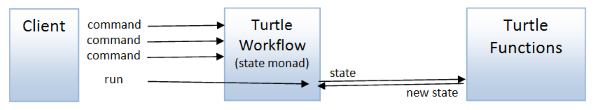### Defining the `turtle` workflow

The core turtle functions that we defined at the very beginning follow the same "shape" as many other state-transforming functions, an input plus the turtle state, and the output plus the turtle state.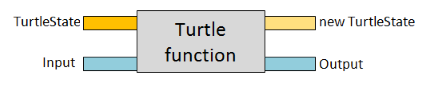(It's true that, so far. we have not had any useable output from the turtle functions, but in a later example we will see this output being used to make decisions.)

There is a standard way to deal with these kinds of functions -- the "state monad".

Let's look at how this is built.

First, note that, thanks to currying, we can recast a function in this shape into two separate one-parameter functions: processing the input generates another function that in turn has the state as the parameter: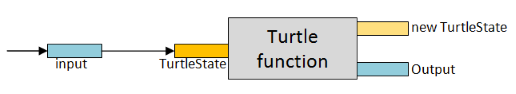We can then think of a turtle function as something that takes an input and returns a new function, like this: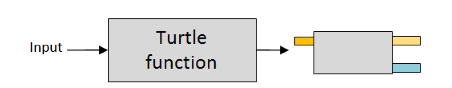In our case, using `TurtleState` as the state, the returned function will look like this:

``````TurtleState -> 'a * TurtleState
``````

Finally, to make it easier to work with, we can treat the returned function as a thing in its own right, give it a name such as `TurtleStateComputation`:In the implementation, we would typically wrap the function with a single case discriminated union like this:

``````type TurtleStateComputation<'a> =
TurtleStateComputation of (Turtle.TurtleState -> 'a * Turtle.TurtleState)
``````

So that is the basic idea behind the "state monad". However, it's important to realize that a state monad consists of more than just this type -- you also need some functions ("return" and "bind") that obey some sensible laws.

I won't define the `returnT` and `bindT` functions here, but you can see their definitions in the full source.

We need some additional helper functions too. (I'm going to add a `T` for Turtle suffix to all the functions).

In particular, we need a way to feed some state into the `TurtleStateComputation` to "run" it:

``````let runT turtle state =
// pattern match against the turtle
// to extract the inner function
let (TurtleStateComputation innerFn) = turtle
// run the inner function with the passed in state
innerFn state
``````

Finally, we can create a `turtle` workflow, which is a computation expression that makes it easier to work with the `TurtleStateComputation` type:

``````// define a computation expression builder
type TurtleBuilder() =
member this.Return(x) = returnT x
member this.Bind(x,f) = bindT f x

// create an instance of the computation expression builder
let turtle = TurtleBuilder()
``````

### Using the Turtle workflow

To use the `turtle` workflow, we first need to create "lifted" or "monadic" versions of the turtle functions:

``````let move dist =
toUnitComputation (Turtle.move log dist)
// val move : Distance -> TurtleStateComputation<unit>

let turn angle =
toUnitComputation (Turtle.turn log angle)
// val turn : Angle -> TurtleStateComputation<unit>

let penDown =
toUnitComputation (Turtle.penDown log)
// val penDown : TurtleStateComputation<unit>

let penUp =
toUnitComputation (Turtle.penUp log)
// val penUp : TurtleStateComputation<unit>

let setColor color =
toUnitComputation (Turtle.setColor log color)
// val setColor : PenColor -> TurtleStateComputation<unit>
``````

The `toUnitComputation` helper function does the lifting. Don't worry about how it works, but the effect is that the original version of the `move` function (`Distance -> TurtleState -> TurtleState`) is reborn as a function returning a `TurtleStateComputation` (`Distance -> TurtleStateComputation<unit>`)

Once we have these "monadic" versions, we can use them inside the `turtle` workflow like this:

``````let drawTriangle() =
// define a set of instructions
let t = turtle {
do! move 100.0
do! turn 120.0<Degrees>
do! move 100.0
do! turn 120.0<Degrees>
do! move 100.0
do! turn 120.0<Degrees>
}

// finally, run them using the initial state as input
runT t initialTurtleState
``````

The first part of `drawTriangle` chains together six instructions, but importantly, does not run them. Only when the `runT` function is used at the end are the instructions actually executed.

The `drawPolygon` example is a little more complicated. First we define a workflow for drawing one side:

``````let oneSide = turtle {
do! move 100.0
do! turn angleDegrees
}
``````

But then we need a way of combining all the sides into a single workflow. There are a couple of ways of doing this. I'll go with creating a pairwise combiner `chain` and then using `reduce` to combine all the sides into one operation.

``````// chain two turtle operations in sequence
let chain f g  = turtle {
do! f
do! g
}

// create a list of operations, one for each side
let sides = List.replicate n oneSide

// chain all the sides into one operation
let all = sides |> List.reduce chain
``````

Here's the complete code for `drawPolygon`:

``````let drawPolygon n =
let angle = 180.0 - (360.0/float n)
let angleDegrees = angle * 1.0<Degrees>

// define a function that draws one side
let oneSide = turtle {
do! move 100.0
do! turn angleDegrees
}

// chain two turtle operations in sequence
let chain f g  = turtle {
do! f
do! g
}

// create a list of operations, one for each side
let sides = List.replicate n oneSide

// chain all the sides into one operation
let all = sides |> List.reduce chain

// finally, run them using the initial state
runT all initialTurtleState
``````

### Advantages and disadvantages of the `turtle` workflow

• The client code is similar to imperative code, but preserves immutability.
• The workflows are composable -- you can define two workflows and then combine them to create another workflow.

• Coupled to a particular implementation of the turtle functions.
• More complex than tracking state explicitly.
• Stacks of nested monads/workflows are hard to work with.

As an example of that last point, let's say we have a `seq` containing a `result` workflow containing a `turtle` workflow and we want to invert them so that the `turtle` workflow is on the outside. How would you do that? It's not obvious!

The source code for this version is available here.

## 9: Batch processing using command objects

Another batch-oriented approach is to reuse the `TurtleCommand` type in a new way. Instead of calling functions immediately, the client creates a list of commands that will be run as a group.

When you "run" the list of commands, you can just execute each one in turn using the standard Turtle library functions, using `fold` to thread the state through the sequence.And since all the commands are run at once, this approach means that there is no state that needs to be persisted between calls by the client.

Here's the `TurtleCommand` definition again:

``````type TurtleCommand =
| Move of Distance
| Turn of Angle
| PenUp
| PenDown
| SetColor of PenColor
``````

To process a sequence of commands, we will need to fold over them, threading the state through, so we need a function that applies a single command to a state and returns a new state:

``````/// Apply a command to the turtle state and return the new state
let applyCommand state command =
match command with
| Move distance ->
move distance state
| Turn angle ->
turn angle state
| PenUp ->
penUp state
| PenDown ->
penDown state
| SetColor color ->
setColor color state
``````

And then, to run all the commands, we just use `fold`:

``````/// Run list of commands in one go
let run aListOfCommands =
aListOfCommands
|> List.fold applyCommand Turtle.initialTurtleState
``````

### Running a batch of Commands

To draw a triangle, say, we just create a list of the commands and then run them:

``````let drawTriangle() =
// create the list of commands
let commands = [
Move 100.0
Turn 120.0<Degrees>
Move 100.0
Turn 120.0<Degrees>
Move 100.0
Turn 120.0<Degrees>
]
// run them
run commands
``````

Now, since the commands are just a collection, we can easily build bigger collections from smaller ones.

Here's an example for `drawPolygon`, where `drawOneSide` returns a collection of commands, and that collection is duplicated for each side:

``````let drawPolygon n =
let angle = 180.0 - (360.0/float n)
let angleDegrees = angle * 1.0<Degrees>

// define a function that draws one side
let drawOneSide sideNumber = [
Move 100.0
Turn angleDegrees
]

// repeat for all sides
let commands =
[1..n] |> List.collect drawOneSide

// run the commands
run commands
``````

• Simpler to construct and use than workflows or monads.
• Only one function is coupled to a particular implementation. The rest of the client is decoupled.

• Batch oriented only.
• Only suitable when control flow is not based on the response from a previous command. If you do need to respond to the result of each command, consider using the "interpreter" approach discussed later.

The source code for this version is available here.

## Interlude: Conscious decoupling with data types

In three of the examples so far (the agent, functional dependency injection and batch processing) we have used a `Command` type -- a discriminated union containing a case for each API call. We'll also see something similar used for the event sourcing and interpreter approaches in the next post.

This is not an accident. One of the differences between object-oriented design and functional design is that OO design focuses on behavior, while functional design focuses on data transformation.

As a result, their approach to decoupling differs too. OO designs prefer to provide decoupling by sharing bundles of encapsulated behavior ("interfaces") while functional designs prefer to provide decoupling by agreeing on a common data type, sometimes called a "protocol" (although I prefer to reserve that word for message exchange patterns).

Once that common data type is agreed upon, any function that emits that type can be connected to any function that consumes that type using regular function composition.

You can also think of the two approaches as analogous to the choice between RPC or message-oriented APIs in web services, and just as message-based designs have many advantages over RPC, so the data-based decoupling has similar advantages over the behavior-based decoupling.

Some advantages of decoupling using data include:

• Using a shared data type means that composition is trivial. It is harder to compose behavior-based interfaces.
• Every function is already "decoupled", as it were, and so there is no need to retrofit existing functions when refactoring. At worst you might need to convert one data type to another, but that is easily accomplished using... moar functions and moar function composition!
• Data structures are easy to serialize to remote services if and when you need to split your code into physically separate services.
• Data structures are easy to evolve safely. For example, if I added a sixth turtle action, or removed an action, or changed the parameters of an action, the discriminated union type would change and all clients of the shared type would fail to compile until the sixth turtle action is accounted for, etc. On the other hand, if you didn't want existing code to break, you can use a versioning-friendly data serialization format like protobuf. Neither of these options are as easy when interfaces are used.